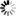# The University of Dodoma Library

Normal view MARC view ISBD view

# Foundation mathematics for the physical sciences / K.F. Riley, M.P. Hobson.

Material type:TextPublisher: Cambridge ; New York : Cambridge University Press, 2011Description: xii, 721 p. : ill. ; 26 cm.ISBN: 9780521192736 (hardback).DDC classification: 510 Other classification: SCI040000 Summary: "This tutorial-style textbook develops the basic mathematical tools needed by first and second year undergraduates to solve problems in the physical sciences. Students gain hands-on experience through hundreds of worked examples, self-test questions and homework problems. Each chapter includes a summary of the main results, definitions and formulae. Over 270 worked examples show how to put the tools into practice. Around 170 self-test questions in the footnotes and 300 end-of-section exercises give students an instant check of their understanding. More than 450 end-of-chapter problems allow students to put what they have just learned into practice. Hints and outline answers to the odd-numbered problems are given at the end of each chapter. Complete solutions to these problems can be found in the accompanying Student Solutions Manual. Fully-worked solutions to all problems, password-protected for instructors, are available at www.cambridge.org/foundation"--
Tags from this library: No tags from this library for this title.Average rating: 0.0 (0 votes)
Item type Current location Call number Copy number Status Date due BarcodeBooks Library, College of Natural and Mathematical Sciences (CNMS)
Special Reserve
QA37.3 .R56 2011 (Browse shelf) 1-1 Available 1828260

Includes index.

"This tutorial-style textbook develops the basic mathematical tools needed by first and second year undergraduates to solve problems in the physical sciences. Students gain hands-on experience through hundreds of worked examples, self-test questions and homework problems. Each chapter includes a summary of the main results, definitions and formulae. Over 270 worked examples show how to put the tools into practice. Around 170 self-test questions in the footnotes and 300 end-of-section exercises give students an instant check of their understanding. More than 450 end-of-chapter problems allow students to put what they have just learned into practice. Hints and outline answers to the odd-numbered problems are given at the end of each chapter. Complete solutions to these problems can be found in the accompanying Student Solutions Manual. Fully-worked solutions to all problems, password-protected for instructors, are available at www.cambridge.org/foundation"--

There are no comments for this item.